### Home > CALC > Chapter Ch3 > Lesson 3.1.1 > Problem3-15

3-15.
1. Remember the conjecture developed in problem 3-5 and find the slope function, f ′(x), for each of the following functions. Homework Help ✎

1. f(x) = x9

2. f(x) = x13

3. f(x) = 2x

4. f(x) = 6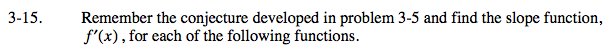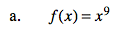BASIC CONJECTURE that you should have made during class:The slope function is one-degree lower than the original function.

ADVANCED CONJECTURE that you might have made during class:The slope function is one degree lower than the original function AND stretched by the degree of the original function.

slope function = 9x8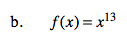Refer to hint for part (a).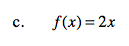This one does not require a conjecture - an Algebra I student could do it! This is the equation of a straight line. What is its slope?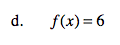Refer to hint for part (c).